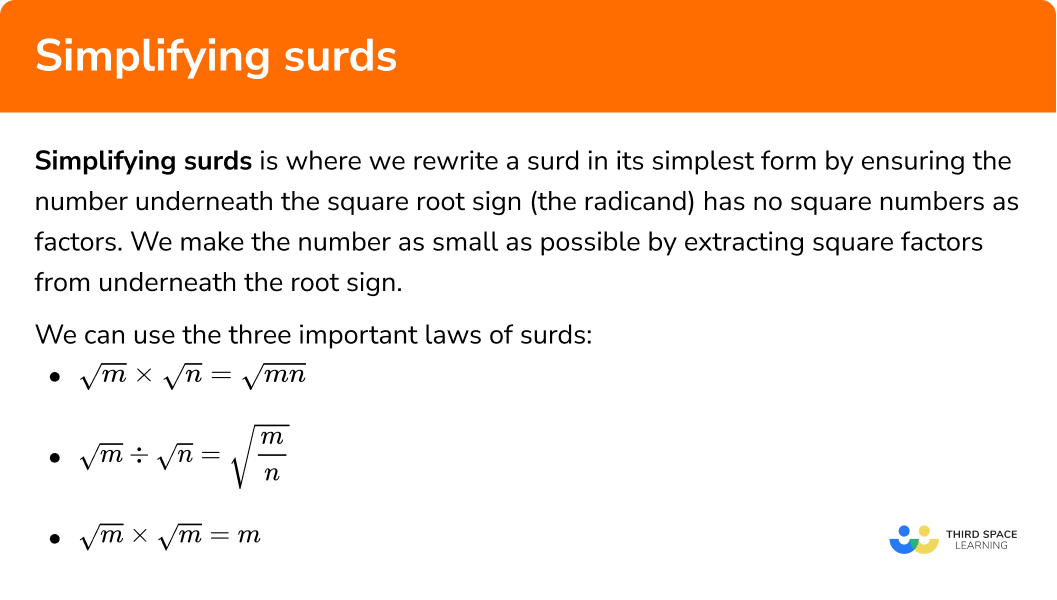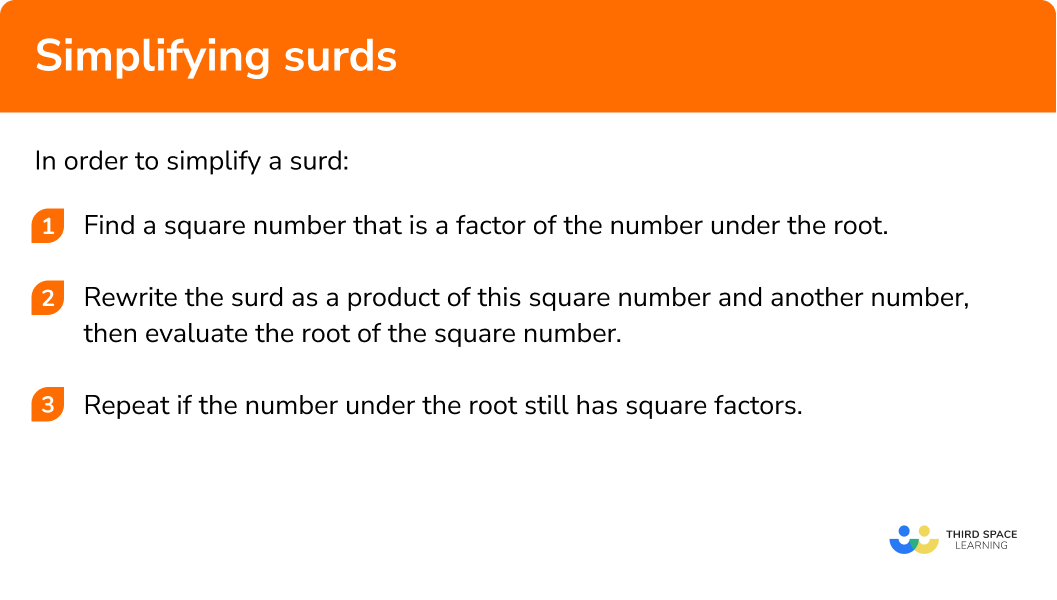GCSE Maths Number Surds

Simplifying surds

# Simplifying Surds

Here we will learn about simplifying surds for GCSE maths including the definition of a surd, the rules of surds, and how to write surds in their simplest form.

There are also simplifying surds worksheets based on Edexcel, AQA and OCR exam questions, along with further guidance on where to go next if you’re still stuck.

## What is simplifying surds?

Simplifying surds is where we rewrite a surd in its simplest form by ensuring the number underneath the root sign (this number is called the radicand) has no square numbers as factors. We make the number as small as possible by extracting square factors from underneath the root sign.

We can use the three important laws of surds, which can be derived from the laws of indices (powers) to help us to simplify surds:

$\sqrt{m} \times \sqrt{n}=\sqrt{m n}$

$\sqrt{m} \div \sqrt{n}=\sqrt{\frac{m}{n}}$

$\sqrt{m} \times \sqrt{m}=m$

E.g.

\begin{aligned} \sqrt{20} &=\sqrt{4 \times 5} \\ &=\sqrt{4} \times \sqrt{5} \\ &=2 \times \sqrt{5} \\ &=2 \sqrt{5} \end{aligned}

### What is simplifying surds?## How to simplify surds

In order to simplify a surd:

1. Find a square number that is a factor of the number under the root.
2. Rewrite the surd as a product of this square number and another number, then evaluate the root of the square number.
3. Repeat if the number under the root still has square factors.

### How to simplify surds## Simplifying surds examples

### Example 1: A simple integer

Simplify:

$\sqrt{8}$

1. Find a square number that is a factor of the number under the root.

Square numbers are 1, 4, 9, 16, 25, …

4 is a factor of 8 (because 4 × 2 = 8).

2Rewrite the surd as a product of this square number and another number, then evaluate the root of the square number.

\begin{aligned} \sqrt{8} &=\sqrt{4 \times 2} \\ &=\sqrt{4} \times \sqrt{2} \\ &=2 \times \sqrt{2} \\ &=2 \sqrt{2} \end{aligned}

3Repeat if the number under the root still has square factors.

In this case, there are no square numbers that are factors of 2, so the surd is fully simplified.

$\sqrt{8}=2 \sqrt{2}$

### Example 2: A simple integer

Simplify:

$\sqrt{45}$

Square numbers are 1, 4, 9, 16, 25, …

9 is a factor of 45 (because 9 × 5 = 45).

\begin{aligned} \sqrt{45} &=\sqrt{9 \times 5} \\ &=\sqrt{9} \times \sqrt{5} \\ &=3 \times \sqrt{5} \\ &=3 \sqrt{5} \end{aligned}

In this case, there are no square numbers that are factors of 5, so the surd is fully simplified.

$\sqrt{45}=3 \sqrt{5}$

### Example 3: A larger integer

Simplify:

$\sqrt{240}$

Square numbers are 1, 4, 9, 16, 25, …

4 is a factor of 240 (because 4 × 60 = 240).

\begin{aligned} \sqrt{240} &=\sqrt{4 \times 60} \\ &=\sqrt{4} \times \sqrt{60} \\ &=2 \times \sqrt{60} \\ &=2 \sqrt{60} \end{aligned}

$\sqrt{240}=2\sqrt{60}$

In this case, 4 is a factor of 60 and also a square number, so the surd is not yet fully simplified. We repeat the process, rewriting 60 as the product 4 × 15.

\begin{aligned} \sqrt{240} &=2 \sqrt{60} \\ &=2 \sqrt{4 \times 15} \\ &=2 \times \sqrt{4} \times \sqrt{15} \\ &=2 \times 2 \times \sqrt{15} \end{aligned}

There are no square numbers that are factors of 15, so we cannot reduce the radicand any further.

To finish off, work out the product of the integers in front of the root (2 × 2 = 4).

The final answer, in its simplest form, is

$\sqrt{240}=4 \sqrt{15}$

Note that you could have completed this simplification in one step by spotting that 16 is a square factor of 240, as follows:

\begin{aligned} \sqrt{240} &=\sqrt{16 \times 15} \\ &=\sqrt{4} \times \sqrt{15} \\ &=4 \sqrt{15} \end{aligned}

This method is clearly more efficient, but it’s not always easy to spot large square factors; in this case, you will probably find it easier to work in stages.

### Example 4: A larger integer

Simplify:

$\sqrt{108}$

Square numbers are 1, 4, 9, 16, 25, …

9 is a factor of 108 (because 9 × 12 = 108).

\begin{aligned} \sqrt{108} &=\sqrt{9 \times 12} \\ &=\sqrt{9} \times \sqrt{12} \\ &=3 \times \sqrt{12} \\ &=3 \sqrt{12} \end{aligned}

$\sqrt{108}=3 \sqrt{12}$

In this case, 4 is a factor of 12 and also a square number, so the surd is not yet fully simplified. We repeat the process, rewriting 12 as the product 4 × 3.

\begin{aligned} \sqrt{108} &=3 \sqrt{12} \\ &=3 \sqrt{4 \times 3} \\ &=3 \times \sqrt{4} \times \sqrt{3} \\ &=3 \times 2 \times \sqrt{3} \end{aligned}

There are no square numbers that are factors of 3, so we cannot reduce the radicand any further.

To finish off, work out the product of the integers in front of the root (3 × 2 = 6).

The final answer in its simplest form is

$\sqrt{108}=6 \sqrt{3}$

### Example 5: Strategies for very large integers

Simplify:

$\sqrt{720}$

16 is a factor of 720 (because 16 × 45 = 720).

\begin{aligned} \sqrt{720} &=\sqrt{16 \times 45} \\ &=\sqrt{16} \times \sqrt{45} \\ &=4 \times \sqrt{45} \\ &=4 \sqrt{45} \end{aligned}

$\sqrt{720}=4 \sqrt{45}$

In this case, 9 is a factor of 45 and also a square number, so the surd is not yet fully simplified. We repeat the process, rewriting 45 as the product 9 × 5.

\begin{aligned} \sqrt{720} &=4 \sqrt{45} \\ &=4 \sqrt{9 \times 5} \\ &=4 \times \sqrt{9} \times \sqrt{5} \\ &=4 \times 3 \times \sqrt{5} \end{aligned}

There are no square numbers that are factors of 5, so we cannot reduce the radicand any further.

To finish off, work out the product of the integers in front of the root (4 × 3 = 12).

The final answer in its simplest form is

$\sqrt{720}=12 \sqrt{5}$

### The prime factor tree method for larger numbers

This is an alternative method for simplifying surds that is very effective for larger numbers. It is similar to, and based on, prime factor trees.

10 and 72 are a factor pair with a product of 720.

2 and 5 are primes, and 4 and 9 are squares.

(Note that we could also stop at 36 which is also square but going further, to make the numbers smaller, makes them easier to deal with.)

Square root both the 4 and the 9 to get 2 and 3 respectively.

$\sqrt{2} \times \sqrt{2}=\sqrt{4}=2$

Finally, 2 × 3 × 2 = 12, so the answer is

$12 \sqrt{5}$

### Example 6: A partially simplified surd expression

Simplify:

$3 \times 4 \sqrt{54}$

Remember that this means

$3 \times 4 \times \sqrt{54}$

Or

$12 \times \sqrt{54}$

So all we need to do is deal with the root 54 as with the previous examples.

9 is a factor of 54 (because 9 × 6 = 54).

\begin{aligned} 12 \times \sqrt{54} &=12 \times \sqrt{9 \times 6} \\ &=12 \times \sqrt{9} \times \sqrt{6} \\ &=12 \times 3 \times \sqrt{6} \\ &=36 \sqrt{6} \end{aligned}

In this case, there are no square numbers that are factors of 6, so the surd is fully simplified.

$3 \times 4 \sqrt{54}=36 \sqrt{6}$

### Common misconceptions

• Incorrectly rewriting the number under the square root sign (the radicand) as a product of any two factors

One of these factors must be a square number in order for you to be able to simplify the surd.

• Not simplifying fully

Always check that there are no square factors of the number under the root.

Simplifying surds is part of our series of lessons to support revision on surds. You may find it helpful to start with the main surds lesson for a summary of what to expect, or use the step by step guides below for further detail on individual topics. Other lessons in this series include:

### Practice simplifying surds questions

1. Simplify: \sqrt{9}

\sqrt{3}3981The square root of 9 is 3 (this is a rational number, so the square root of 9 is not a surd).

2. Simplify: \sqrt{20}

2 \sqrt{10}4 \sqrt{5}5 \sqrt{4}2 \sqrt{5}4 is the largest square factor of 20,  (4\times5=20) . The square root of 4 is 2 .

3. Simplify: \sqrt{15}

3 \sqrt{5}2 \sqrt{15}\sqrt{15}5 \sqrt{3}There are no square numbers that are factors of 15 , so this surd is already simplified as far as possible.

4. Simplify: \sqrt{150}

6\sqrt{5}\sqrt{150}10\sqrt{15}5\sqrt{6}25 is the largest square factor of 150, (25\times6=150) . The square root of 25 is 5 .

5. Simplify: \sqrt{368}

4\sqrt{23}4\sqrt{92}2\sqrt{23}2\sqrt{92}16 is the largest square factor of 368,  (16\times23=368) . The square root of 16 is 4 .

6. Simplify: 3\sqrt{280}

12\sqrt{70}6\sqrt{70}6\sqrt{140}9\sqrt{140}4 is the largest square factor of 280, (4\times70=280) . The square root of 4 is 2 , so \sqrt{280}=2 \sqrt{70} . Finally, multiply by the 3 to get 6\sqrt{70} .

### Simplifying surds GCSE questions

1. Simplify
\sqrt{84}

(2 marks)

\sqrt{84}=\sqrt{4} \times \sqrt{21}

(1)

2 \sqrt{21}

(1)

2. Simplify fully
\sqrt{540}

(2 marks)

\sqrt{540}=\sqrt{36} \times \sqrt{15} \text { or } \sqrt{540}=\sqrt{4} \times \sqrt{135}

(1)

6 \sqrt{15}

(1)

3. \sqrt{912}=4\sqrt{k}
where k is an integer. Find the value of k .

(2 marks)

\sqrt{912}=\sqrt{16}\times \sqrt{k}
or sight of 912\div16

(1)

4 \sqrt{57}
so k=57

(1)

4. Simplify fully
5 \sqrt{80}

(2 marks)

5 \sqrt{80}=5\times\sqrt{5}\times\sqrt{16}

(1)

20\sqrt{5}

(1)

## Learning checklist

You have now learned how to:

• Identify a surd as an irrational root
• Simplify surds

## Still stuck?

Prepare your KS4 students for maths GCSEs success with Third Space Learning. Weekly online one to one GCSE maths revision lessons delivered by expert maths tutors.

Find out more about our GCSE maths tuition programme.